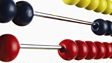A maths quiz show using a number rectangle to show the rules of addition. Look at the number rectangle. Which number is one more than another number? Numbers on the same row have a difference of one. Can you find a number that is ten more than another number? When a number is directly underneath another number, then it is ten more. What is the easy way to find a number 11 more than another number? If you move down a row then go one place to the right, it is 11 more. What is the easy way to add 22?This clip is from:
The Maths Channel, Year 2: Place Value